# Formula for curvature of dual connection

Suppose$M$ is a differential manifold,$E$ is a vector bundle over$M$, and$\nabla$ is a connection on$E$. Suppose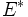$E^*$ is the dual bundle and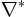$\nabla^*$ is the dual connection to$\nabla$. If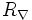$R_\nabla$ and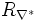$R_{\nabla^*}$ denote respectively the Riemann curvature tensors of$\nabla$ and$\nabla^*$, then we have: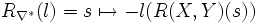$R_{\nabla^*}(l) = s \mapsto -l(R(X,Y)(s))$.